## MP Board Class 12th Maths Important Questions Chapter 6 Application of Derivatives

### Application of Derivatives Important Questions

Application of Derivatives Objective Type Questions:

Question 1.

Question 1.
The rate of change of the area of a circle with respect to its radius r when r = 5 is:
(a) 10 π
(b) 12 π
(c) 8 π
(d) 11 π.
(b) 12 π

Question 2.
Tangent line of a curve y2 = 4x at y = x + 1 is:
(a) (1,2)
(b) (2, 1)
(c) (1, -2)
(d) (- 1, 2).
(a) (1,2)

Question 3.
Approximate change in the volume of a cube of side x metre caused by increasing the side by 2%:
(a) 0.03 x3
(b) 0.02 x3
(c) 0.06 x3
(d) 0.09 x3
(c) 0.06 x3Question 4.
Point on the curve x2 = 2y which lie minimum distance from the point (0,5):
(a) (2$$\sqrt{2}$$,4)
(b) (2$$\sqrt{2}$$,0)
(c) (0, 0)
(d) (2, 2).
(a) (2$$\sqrt{2}$$,4)

Question 5.
Minimum value of the function f(x) = x4 – x2 – 2x + 6:
(a) 6
(b) 4
(c) 8
(d) None of these.
(b) 4

Question 2.
Fill in the blanks:

1. The function f(x) = cosx, for 0 ≤ x ≤ π is …………………………..
2. The radius of circular plate is increasing at the rate of 0.2 cm /sec. when r = 10, then the rate of change of the area of the plate is ………………………………
3. The function y = x(5 – x) is maximum at x is equal to ……………………………
4. The minimum value of 2x + 3y is ………………………….. when xy = 6.
5. The maximum value of sin x + cos x is ……………………………
6. If line y = mx+ 1 is a tangent line to the curve y2 = 4x. Then value of m will be ……………………………..
7. Slope of the tangent to the curve y = x2 at the point (1, 1) is ………………………….
8. Using differential the approximate value of $$\sqrt{0.6}$$ is …………………………….

1. Decreasing
2. 4πcm2/sec
3. $$\frac{5}{2}$$
4. 12
5. $$\sqrt{2}$$
6. 1,
7. 2,
8. 0.8.Question 3.
Write True/False:

1. For all real values of x, the function f(x) = ex – e-x is increasing function.
2. If the length of equal sides of an isosceles traingle be x then its maximum area will be $$\frac{1}{2}$$ x2
3. Function f(x) = 3x2 – 4x is increasing in the interval (- ∞, $$\frac{2}{3}$$ )
4. Function f(x) = x – cot x is always decreasing.
5. Equation of the normal of the curve y = ex at point (0, 1) is x + y = l.

1. True
2. True
3. False
4. False
5. True.

Application of Derivatives Short Answer Type Questions

Question 1.
The radius of a circle increases at the rate of 2cm/sec. At what rate the area increases when radius is 10cm?
Solution:
Given:
$$\frac { dr }{ dt }$$ = 2cm/sec
Let the area of circle be A
Then,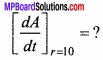∵ Area of circle A = πr2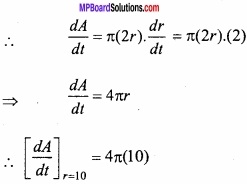= 40π sq.cm/secondQuestion 2.
The radius of air bubble is increasing at the rate of 1/2 cm per second. At what rate the volume of the air bubble is increasing when the radius is 1 cm?
Solution:
Let the radius of air bubble be r.
∴ Volume V = $$\frac{4}{3}$$ πr3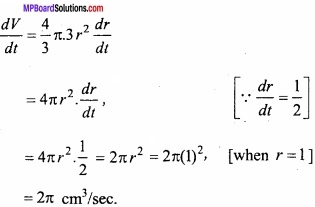Question 3.
The radius of a balloon is increasing at the rate of 10 cm/sec? At what rate surface area of the balloon is increasing when radius is 15cm?
Solution:
Let r be the radius of balloon at any time t and its surface be x then
A = 4πr2
Differentiating eqn.(1) w.r.t. t,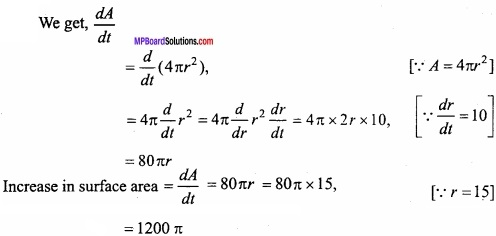Hence, when radius of ballon is 15cm, then its area is increasing at rate of 1200π cm/sec.

Question 4.
Find those intervals in which the function f(x) = 2x3 – 15x2 + 36x + 1 is increasing or decreasing?
Solution:
f(x) = 2x3 – 15x2 + 36x + 1
⇒ f'(x) = 6x2 – 30x + 36
= 6(x2 – 5x + 6)
= 6(x – 2) (x – 3)
For increasing function of f(x),
f'(x) > 0
or (x – 2) (x – 3) > 0
⇒ x – 2 > 0 and x – 3 > 0
⇒ x > 2 and x < 3 ⇒ x > 3
Clearly the function is increasing in interval (3, ∞)
Again, (x – 2) (x – 3) > 0
or x – 2 < 0 and x – 3 < 0
⇒ x < 2 and x < 3
⇒ x < 2
Clearly the function is increasing in interval (-∞, 2)
Hence, the function is increasing in the interval (-∞, 2) ∪ (3, ∞)
Again for decreasing function of f(x),
f'(x) < 0
⇒ (x – 2) (x – 3) < 0
or x – 2 < 0 and x – 3 > 0
⇒ x < 2 and x > 3, which is impossible
or x – 2 > 0 and x – 3 < 0 ⇒ x > 2 and x < 3
⇒ 2 < x < 3
Hence, f(x) is decreasing function in the interval (2, 3).Question 5.
(A) If x + y = 8, then find maximum value of xy?
Solution:
Let P = xy
⇒ x + y = 8
⇒ y = 8 – x
∴P = x(8 – x) = 8x – x2
⇒ $$\frac { dP }{ dx }$$ = 8 – 2x
⇒ $$\frac { d^{ 2 }P }{ dx^{ 2 } }$$ = -2
For maximum or minimum value
8 – 2x = 0
⇒ x = 4
Now, x = 4 then $$\frac { d^{ 2 }P }{ dx^{ 2 } }$$ = -2, which is negative
∴ at x = 4, then y = 4
Maximum value of P, when x = 4, y = 4
= 4 × 4 = 16.

(B) If x + y = 10 then find maximum value of xy?
Solution:
Solve like Q.No.5(A).

Question 6.
The radius of a circle increases at the rate of 3cm/sec. At what rate the area increases when the radius is 10cm?
Solution:
Let r be the radius and A be the area of circle then,
A = πr2
$$\frac { dA }{ dt }$$ = rate of change of area = ?
⇒ $$\frac { dA }{ dt }$$ = 2πr $$\frac { dr }{ dt }$$
⇒ $$\frac { dA }{ dt }$$ = 2π.(10).3
= 60π cm2/second.Question 7.
The volume of a cube is increasing at the rate of 9 cm3/sec? If the edge of cube is 10cm then at what rate the surface area of cube is increasing? (NCERT)
Solution:
Let the edge of cube = a dm
Volufne = V= a3
Surface area of cube = s = 6a2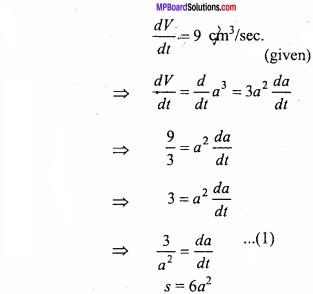Surface area of cube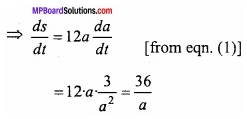When a = 10cm, then $$\frac { ds }{ dt }$$ = $$\frac { 36 }{ 10 }$$ = 3.6 cm2/sec.

Question 8.
(A) A man 180 cm high walks away from a lamp post at a rate of 1.2metre per second. If the height of lamp post is 4.5 metre. Find the rate at which the length of his shadow increases?
Solution:
Let AB be the lamp post of the height 4.5 m, PQ be the position of man at time t, CQ = x and BQ = y
drrf

(B) A man of height 2 metre walking away from a lamp post at a rate of 5km/ sec. If the height of the lamp post 6m. Find the rate at which the length of his shadow is increasing? (NCERT)
Solution:
Solve like Q. No.8(A).
[Ans. $$\frac{5}{8}$$ km/sec.]

Question 9.
A ladder 5 metre long is leaning against a wall. The bottom of the ladder is pulled along the ground away from the wall, at the rate of 2 metre per second. How just is its height on the wall decreasing when the foot of the ladder is 4 metre away from the wall? (NCERT)
Solution:
Let at any time t, the bottom of the ladder be at a distance x metre from the wall and the height of the wall bey metre.
QA = xm, OB = ym, AB = 5m (given)
$$\frac { dx }{ dt }$$ = 2m/sec.
In ∆OAB,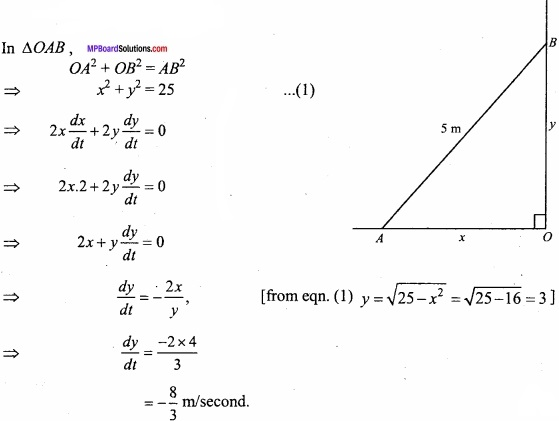Question 10.
Find the intervals in which f(x) = 5x3 + 7x – 13 is increasing or decreasing?
Solution:
Given:
f(x) = 5x2 + 7x – 13 (given)
∴ f'(x) = 10x + 7
If f(x) is increasing,
f'(x) > 0
⇒ 10x + 7 > 0
⇒ x > $$\frac{-7}{10}$$
Hence, f(x) is increasing in interval ( $$\frac{-7}{10}$$ , ∞)
If f(x) os decreasing, then,
f'(x) < 0
⇒ 10x + 7 < 0
⇒ x < $$\frac{-7}{10}$$
∴ f(x) is decreasing in (-∞, $$\frac{-7}{10}$$ ).Question 11.
Find the intervals in which the function f(x) = 2x3 – 24x + 5 is increasing or decreasing?
Solution:
f(x) = 2x3 – 24x + 5
Differentiating w.r.t x,
f'(x) = 6x2 – 24
⇒ f'(x) = 6(x2 – 4)
⇒ f'(x) = 6(x + 2) (x-2) …………………. (2)
(A) If f(x) in is increasing, then
f'(x) > 0
6(x + 2)(x-2) > 0
∴ f(x), x ∈ (-∞,-2) ∪(2, ∞) is increasing.
(B) If f(x) is decreasing,
f'(x) < 0 ⇒ 6(x + 2) (x – 2) < 0
f(x), x ∈ (-2,2) decreasing.

Question 12.
Show that the function f(x) = x – cos x is always increasing?
Solution:
Given function f(x) = x – cos x
∴ f'(x) = 1 – (- sin x)
⇒ f'(x) = 1 + sin x
We know that -1 ≤ x ≤ 1
-1 + 1 ≤ 1 + sin x ≤ 1 + 1
0 ≤ 1 + sin x ≤ 2
Hence f'(x) = 1 + sin x is always positive for all values of x.
∴ f(x) = x – cos x is always increasing.Question 13.
Find the least value of a such that the function given by f(x) = x2 + ax + 1 is strictly increasing on (1, 2)? (NCERT)
Solution:
Given:
f(x) = x2 + ax + 1
∴ f'(x) = 2x + a
When, x ∈ (1,2)
∴ 1 < x < 2
⇒ 2 < 2x < 4
⇒ 2 + a < 2x + a < A + a
⇒ 2 + a < f'(x) < 4 + a Since f(x) is increasing function ∴ f'(x) > 0
⇒ 2 + a > 0 ⇒ a > -2
Hence, the least value of a is -2.

Question 14.
Let I be any interval disjoint from [-1, 1] prove that the function f given by f(x) = x + $$\frac{1}{x}$$ is strictly increasing on I? (NCERT)
Solution:
f(x) = x + $$\frac{1}{x}$$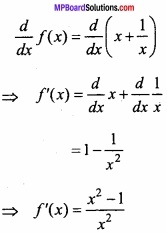x ∈ 1, x ∉ (-1, 1)
x < – 1 0r x > 1
⇒ x2 – 1 > 0
⇒ $$\frac { x^{ 2 }-1 }{ x^{ 2 } }$$ > 0
⇒ f'(x) > 0, x ∈ 1
∴ f(x) is increasing on I. Proved.Question 15.
Find the interval in which the given function is increasing or decreasing:
f(x) = x4 – $$\frac { x^{ 3 } }{ 3 }$$?
Solution:
f(x) = x4 – $$\frac { x^{ 3 } }{ 3 }$$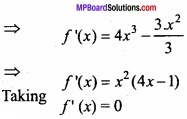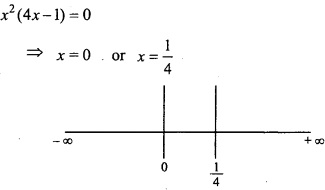Hence, the interval 0 and $$\frac{1}{4}$$ on X – axis is divided in three intervals
(- ∞, 0), (0, $$\frac{1}{4}$$ ), ( $$\frac{1}{4}$$, ∞)
In interval (- ∞, 0)
f'(x) = x2 (4x – 1),
⇒ f'(x) < 0 [x2 = +ve] [4x – 1 > 0]
⇒ f'(x) < 0
∴ In interval (- ∞, 0) the function f(x) decreasing
In interval (0, $$\frac{1}{4}$$ ):
f'(x) = x2 (4x – 1),
⇒ f'(x) > 0 [∵ x2 = positive] [4x – 1 > 0]
∴ In interval (0, $$\frac{1}{4}$$ ) f(x) is decreasing
In interval ( $$\frac{1}{4}$$, ∞):
f'(x) = x2 (4x – 1), [∵ x2 = positive] [4x – 1 > 0]
⇒ f'(x) > 0
In interval ( $$\frac{1}{4}$$, ∞) in function f(x) increasing.Question 16.
The perimeter of a rectangle is 100 cm. Find the length of sides of the rectangle for maximum area?
Solution:
Let length of rectangle be x and breadth be y.
∴Perimeter of rectangle = 2(x + y)
⇒ 2x + 2y = 100
⇒ x + y = 50
Let area of rectangle,
A = xy = x(50 – x) = 50x – x2, [from eqn.(1)]
$$\frac { dA }{ dx }$$ = 50 – 2x
and $$\frac { d^{ 2 }A }{ dx^{ 2 } }$$ = -2
For maximum or minimum of A,
$$\frac { dA }{ dx }$$ = 0
⇒ 50 – 2x = 0 or or x = 25
For any value of x, $$\frac { d^{ 2 }A }{ dx^{ 2 } }$$ is -ve
For x = 25, area of rectangle is maximum
Put in eqn.(1),
y = 50 – x = 50 – 25 = 25.Question 17.
Area of a rectangle is 25 sq.cm. Find its length and breadth when its perimeter is minimum?
Solution:
Let the length and breadth of rectangle be x and y units and A be the area.
xy = 25 ……………………….. (1)
Perimeter of rectangle,
P = 2(x + y)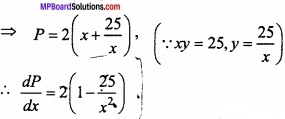For maximum or minimum $$\frac { dP }{ dx }$$ = 0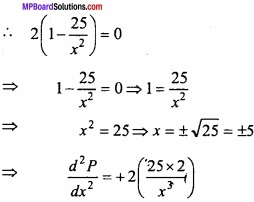Which is positive for x = 5
∴ For Minimum parimeter x = cm.
y = $$\frac{25}{x}$$ = $$\frac{25}{5}$$ = 5 cm.

Question 18.
Find the maximum value of sin x + cos x = $$\sqrt{2}$$? (NCERT)
Solution:
Given:
f(x) = sin x + cos x …………………. (1)
∴f'(x) = cos x – sin x …………………… (2)
and f”(x) = – sinx – cos x …………………….. (3)
For maxima or minima
f'(x) = 0
∴ cos x – sin x = 0
⇒ sin x = cos x
⇒ tan x = 1
∴ x = $$\frac { \pi }{ 4 }$$, $$\frac { 3\pi }{ 4 }$$, $$\frac { 5\pi }{ 4 }$$
Put x = $$\frac { \pi }{ 4 }$$ in eqn (3)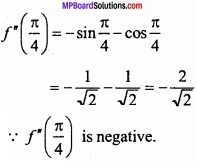Hence, at x = $$\frac { \pi }{ 4 }$$ the given function is maximum. In the same way for x = $$\frac { 3\pi }{ 4 }$$, $$\frac { 5\pi }{ 4 }$$ ………………… the function is maximum.
Put x = $$\frac { \pi }{ 4 }$$ in eqn.(1),
Maximum value f ( $$\frac { \pi }{ 4 }$$ ) = sin $$\frac { \pi }{ 4 }$$ + cos $$\frac { \pi }{ 4 }$$
= $$\frac { 1 }{ \sqrt { 2 } }$$ + $$\frac { 1 }{ \sqrt { 2 } }$$ = $$\frac { 2 }{ \sqrt { 2 } }$$ = $$\sqrt{2}$$. Proved.

Question 19.
Two positive numbers are such that x +y = 60 and xy3 is maximum. Prove (NCERT)
Solution:
Given:
x + y = 60 …………………….. (1)
Let p = xy3
⇒ P = (60 – y). y3
⇒ P = 60y3 – y4
⇒ $$\frac{dp}{dy}$$ = 180 y2 – 4y3 …………………….. (2)
Put $$\frac{dp}{dy}$$ = 0
180 y2 – 4y2 = 0
⇒ 4y2 (45 – y) = 0
∴ y = 45
Differentiating eqn. (2) w.r.t y,
$$\frac { d^{ 2 }p }{ dy^{ 2 } }$$ = 360y – 12y2
= 12 y(30 – y)
Put y = 45,
$$\frac { d^{ 2 }p }{ dy^{ 2 } }$$ = 12 × 45 (30 – 45) = – ve
Hence, at y = 45, p is maximum
From eqn.(1),
x + 45 = 60
⇒ x = 15
∴ The two numbers are 15 and 45.

Question 20.
Find the slope of the tangent to curve y = x3 – x + 1 at the point whose x coordinate is 2? (NCERT)
Solution:
The equation of given curve,
y = x3 – x + 1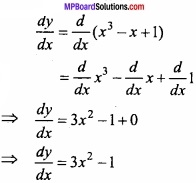Slope of tangent at x = 2,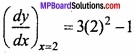= 3 × 4 – 1 = 11.

Question 21.
Find the slope of the tangent to the curve y = $$\frac { x-1 }{ x-2 }$$, x ≠ 2 at x = 10? (NCERT)
Solution:
The equation of given curve: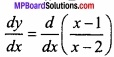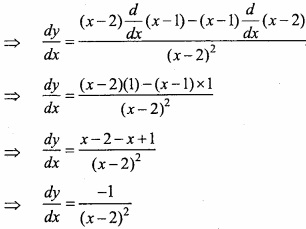At x = 10 slope of tangent is: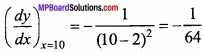Question 22.
Find the point at which the tangent to curve y = x3 – 3x2 – 9x + 7 is parllel to X – axis? (NCERT)
Solution:
Equation of given curve y = x3 – 3x2 – 9x + 7
$$\frac { dy }{ dx }$$ = $$\frac { d }{ dx }$$ (x3 – 3x2 – 9x + 7)
⇒ $$\frac { dy }{ dx }$$ = 3x2 – 6x – 9
= 3[x2 – 2x – 3]
= 3[x2 – 3x + x – 3]
= 3[x(x – 3) + 1(x – 3)]
⇒ $$\frac { dy }{ dx }$$ = 3(x – 3) (x + 1)
Tangent is parllel to X – axis.
∴$$\frac { dy }{ dx }$$ = 0
3 (x – 3) (x + 1) = 0
⇒ x = 3,-1
When x = 3, then y = (3)3 – 3(3)2 – 9 × 3 + 7
y = 27 – 27 – 27 + 7
y = – 20
When x = 3, then y = (-1)3 – 3(-1)2 – 9(-1) + 7
y = – 1 – 3 + 9 + 7
y = 12
Hence, the point at which the tangent is parllel to X – axis are (3, -20) and (-1, 12).Question 23.
Find the equation of the tangent to the parabolas y2 = 4ax at the point (at2, 2at)? (NCERT)
Solution:
Given:
y2 = 4ax ……………………. (1)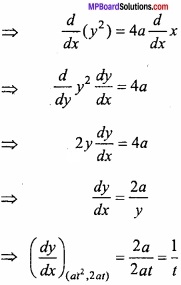Equation of tangent is:
y – y1 = $$\frac { dy }{ dx }$$ (x – x1)
Where x1 = at2, y1 = 2at, $$\frac { dy }{ dx }$$ = $$\frac { 1 }{ t }$$
y – 2at = $$\frac { 1 }{ t }$$ (x – at2)
⇒ yt – 2at2 = x – at2
⇒ x – ty + at2 = 0.

Question 24.
Find the equation of the tangent to the curve x2/3 + y2/3 = 2 at point (1, 1)? (NCERT)
Solution:
Equation of curve:
x2/3 + y2/3 = 2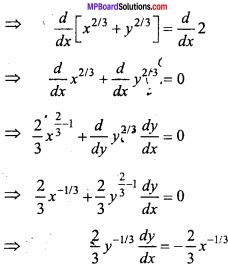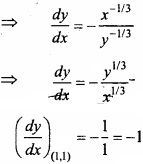Equation of tangent at point (x1, y1) is:y – 1 = – (x – 1)
⇒ y – 1 = – x + 1
⇒ x + y – 2 = 0

Question 25.
Find the equation of tangent to the curve 2y + x2 = 3 at point (1, 1)? (NCERT)
Solution:
Equation of given curve:
2y + x2 = 3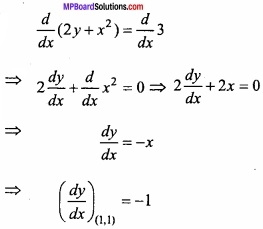Equation of tangent at point (x1, y1) is: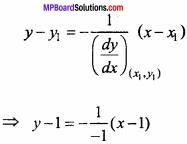⇒ y – 1 = x – 1
⇒ x – y = 0.

Question 26.
(A) Find the equation of tangent to the cure x = cos t, y = sin at t = $$\frac { \pi }{ 4 }$$? (NCERT)
Solution:
Equation of 1st curve:
x = cos t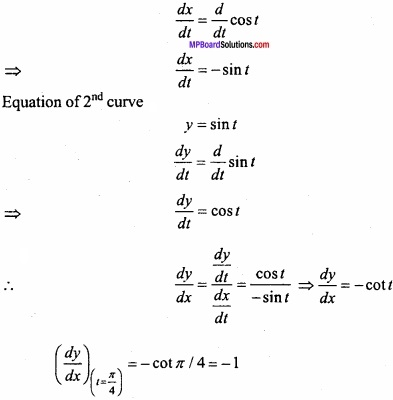x = cos t = cos $$\frac { \pi }{ 4 }$$ = $$\frac { 1 }{ \sqrt { 2 } }$$
y = sin t = sin $$\frac { \pi }{ 4 }$$ = $$\frac { 1 }{ \sqrt { 2 } }$$
Equation of tangent at (x1, y1) is: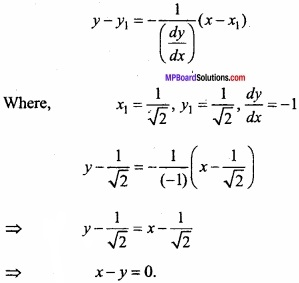(B) Find the equation of tangent and normal to the curve 16x2 + 9y2 = 145 at point (x1, y1), where x1 = 2 and y1 > 0? (CBSE 2018)
Solution:
Given:
16x2 + 9y2 = 145 ……………………. (1)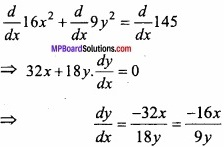Put x = 2 in eqn.(1), we get
16.(2)2 +9y2 =145
⇒ 64 + 9y2 =145
⇒ 9y2 = 145 – 64 = 81
⇒ y2 =9, [y ≠ -3 ∵ y1 > 0]
⇒ y = 3
At point (2, 3)
$$\frac { dy }{ dx }$$ = – $$\frac { 16 }{ 9 }$$. $$\frac { 2 }{ 3 }$$ = – $$\frac { 32 }{ 27 }$$
Equation of tangent of curve (1) at point (2, 3),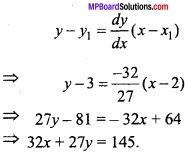and equation of normal of curve (1) at point (2, 3) is,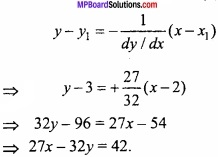Question 27.
Use differential to approximate (25)1/3? (NCERT)
Solution:
Let y = x1/3
Where, x = 27 and ∆x = -2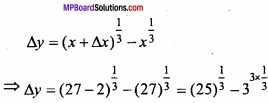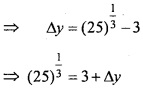∆y is appromimately equal to dy.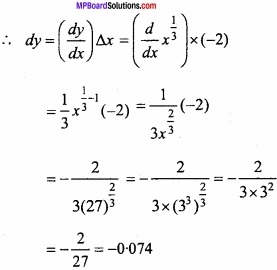Approximately value of (25)1/3
= 3 + ∆y
= 3 – 0.074 = 2.926.

Question 28.
Use differential to approximate $$\sqrt { 36.6 }$$? (NCERT)
Solution:
Let y = $$\sqrt{x}$$, where x = 36 and ∆x = 0.6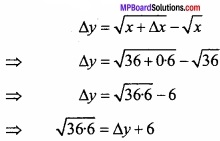∆y is appromimately equal to b,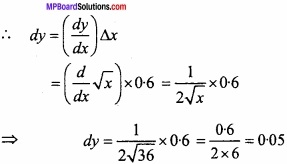Approximate value of $$\sqrt { 36.6 }$$
= ∆y + 6
= 0.05 + 6 = 6.05

Question 29.
Use differential to approximate (15)1/4? (NCERT)
Solution:
Let y = x1/4
Where, x = 16 and ∆x = -1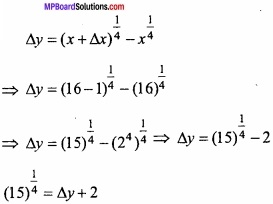∆y is appromimately equal to dy,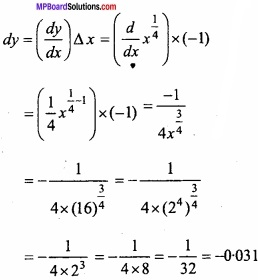Approximately value of (15)1/4
∆y + 2 = 2 – 0.031 = 1.969.

Question 30.
Use differential to approximate (26)1/3? (NCERT)
Solution:
Let y = x1/3
Where, x = 27 and ∆x = -1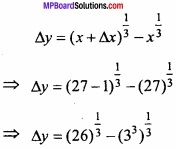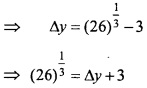∆y is appromimately equal to dy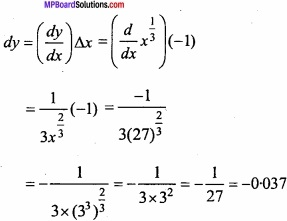Approximate value of (26)1/3
= ∆y + 3
= 3 – 0.037 = 2.963Question 31.
If the radius of the sphere is measured as 9cm with an error 0.03 cm then find the approximate error in calculating its surface area? (NCERT)
Solution:
Let the radius of circle = r
Given:
r = 9 cm, ∆r = 0.03 cm
Area of sphere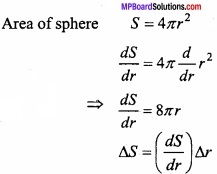= (8πr) × 0.03
= 8π × 9 × 0.03 = 2.16 π cm2
The approximate error in caluculating the surface area is = 2.16 π cm2

Question 32.
If the radius of a sphere is measured as 7m with an error of 0.02m then find the approximate error in calculating its volume?
Solution:
Let the radius of sphere = r
and ∆r be the error in measuring the radius.
r = 7m, ∆r = 0.02 m (given)
Volume of sphere V = $$\frac{4}{3}$$ πr3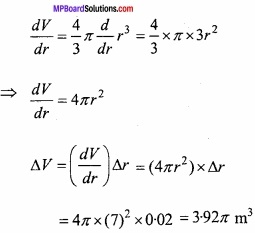The approximate error in calculating the volume is 3.92π m3Question 33.
Find the approximate change in the volume V of a cube of sides x metre caused by increasing the side by 1%? (NCERT)
Solution:
Let x be the sides of cube.
Volume of cube V = x3, ∆x = 1% of x = $$\frac{x}{100}$$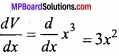Change in volume ∆V = ( $$\frac { dV }{ dx }$$ ) ∆x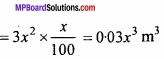Approximate change in volume = 0.03 x3m3.

Question 34.
Find the approximate change in voume V of a cube of side x metre caused by increasing the side by 2%
Solution:
Let x be the side of cube,
∆x = 2% of x = $$\frac { x\times 2 }{ 100 }$$ = 0.02 x
Volume of cube V = x3
$$\frac { dv }{ dx }$$ = $$\frac { d }{ dx }$$ x3 = 3x2
dV = ( $$\frac { dv }{ dx }$$ ) ∆x = 3x2 × 0.02 x = 0.06x3 m3
Thus the approximate change in voume = 0.06 x3m3.